Get instant live expert help with Excel or Google Sheets“My Excelchat expert helped me in less than 20 minutes, saving me what would have been 5 hours of work!”

#### Post your problem and you’ll get expert help in seconds.

Your message must be at least 40 characters
Our professional experts are available now. Your privacy is guaranteed.

# Excel ROMAN Function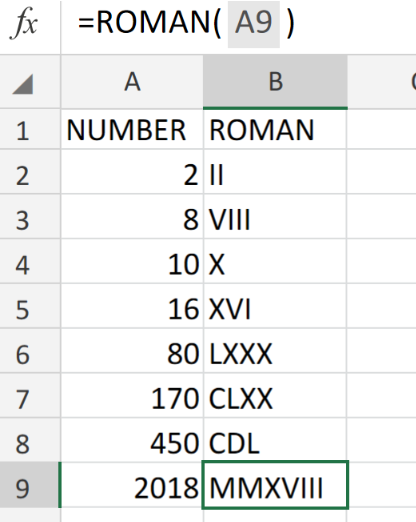Figure 1. of ROMAN Function in Excel.

In this post, we are going to describe the formula syntax of the ROMAN Function in Excel and demonstrate its purpose.

## Generic Formula

`ROMAN(number, [form])`

The ROMAN Function will automatically convert Number Values into Roman numerals.

## How to use the Excel ROMAN Function

We are going to utilize the Excel ROMAN Function by following three simple steps.

1. Arrange the number values we are going to convert into one column of our worksheet. Label a separate column for Excel to generate our results.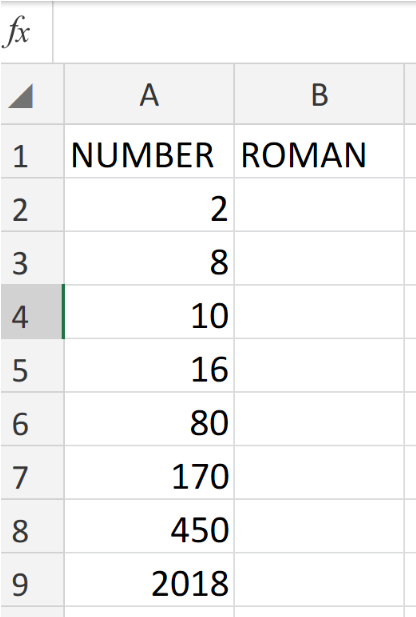Figure 2. of Number Values for Conversion to ROMAN Numerals in Excel.

1. We can now proceed to input the following formula into cell B2 of our worksheet.

`=ROMAN(A2:A5)`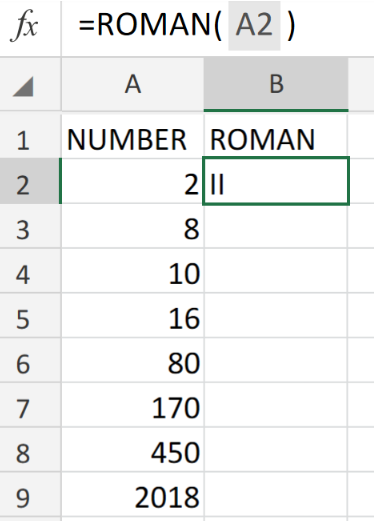Figure 3. of ROMAN Function in Excel.

1. The ROMAN Function will automatically convert the number value found in cell A2 above, into the Roman numeral II. Modify and copy the formula into the other cells in the entire column B to get the desired Roman numeral equivalents.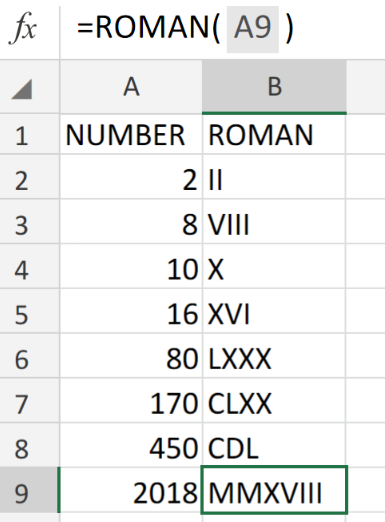Figure 4. of ROMAN Function in Excel.

## Note

• The number values for conversion must be positive.
• The ROMAN Function in Excel will automatically convert Number Values into Roman numerals in the form of text (IV)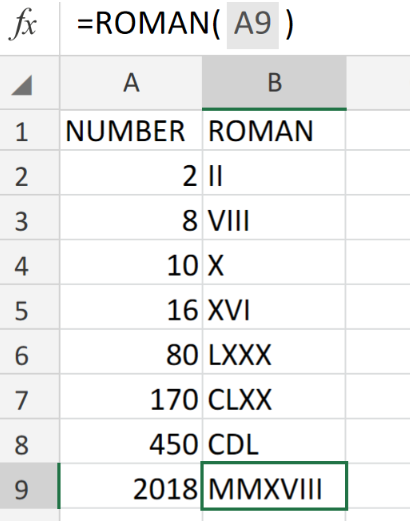Figure 5. of Final Result.

## Instant Connection to an Expert through our Excelchat Service

Our live Excelchat Service is here for you. We have Excel Experts available 24/7 to answer any Excel questions you may have. Guaranteed connection within 30 seconds and a customized solution for you within 20 minutes.